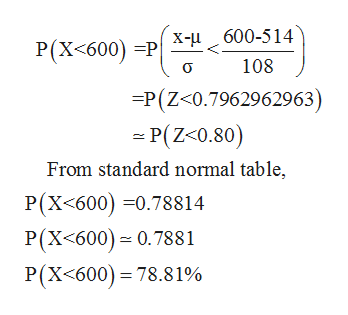# Use the normal distribution of SAT critical reading scores for which the mean is 514and the standard deviation is108. Assume the variable x is normally distributed. What percent of the SAT verbal scores are less than600600​? If 1000 SAT verbal scores are randomly​ selected, about how many would you expect to be greater than575575​?

Question
4 views
Use the normal distribution of SAT critical reading scores for which the mean is 514and the standard deviation is
108. Assume the variable x is normally distributed.

 What percent of the SAT verbal scores are less than 600600​? If 1000 SAT verbal scores are randomly​ selected, about how many would you expect to be greater than 575575​?
check_circle

Step 1

Given data normally distributed with mean of 514 and standard deviation of 108.

Let ‘X’ be a normal distribution random variable.

Step 2

Now, percent of the SAT verbal scores are less than 600 is,help_outlineImage TranscriptioncloseX-u 600-514 P(X<600) =P 108 =P(Z<0.7962962963) - P(Z<0.80) From standard normal table, P(X<600) =0.78814 P(X<600) = 0.7881 P(X<600)= 78.81% fullscreen
Step 3

Therefore, required percent of the SAT verbal scores...

### Want to see the full answer?

See Solution

#### Want to see this answer and more?

Solutions are written by subject experts who are available 24/7. Questions are typically answered within 1 hour.*

See Solution
*Response times may vary by subject and question.
Tagged in

### Statistics